# Determine the new differential reading in a manometer

• Bolter

#### Bolter

Homework Statement
See below
Relevant Equations
pressure = rho x g x h
Not sure how to start off this question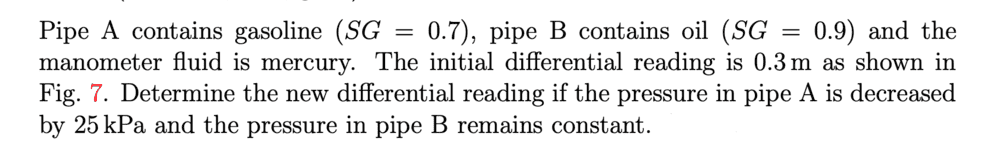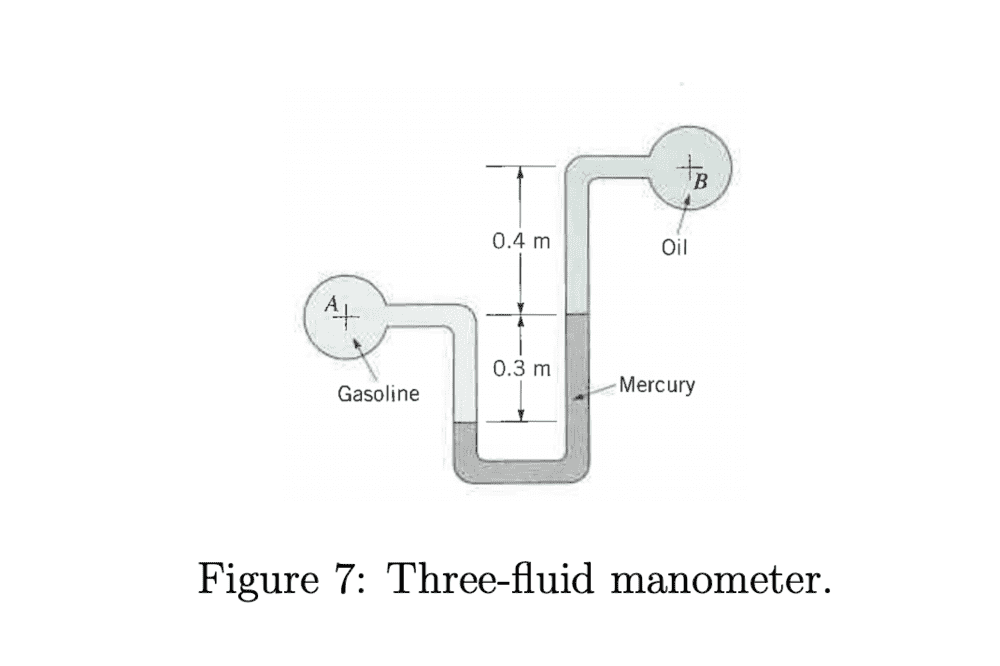I'm confused how to begin if I do not the exact pressure on either pipe A or pipe B

Only thing that I can deduce from this is that if pipe A exerts a smaller pressure than before then the mercury column on the left side would rise i.e. the new manometer reading would have to be less than 0.3m. I can't figure out how to get this new reading however which would be less than 0.3m

Thanks for any help given!

Last edited:

What is the initial difference in pressure between the gasoline and the oil?

What is the initial difference in pressure between the gasoline and the oil?

Is it 25 kPa? How can initial difference in pressure be determined between the 2 fluids if both A & B pressures are not known?

What is the initial difference in pressure between the gasoline and the oil?

Wait the initial pressure difference would be 41496.3 pa when doing this?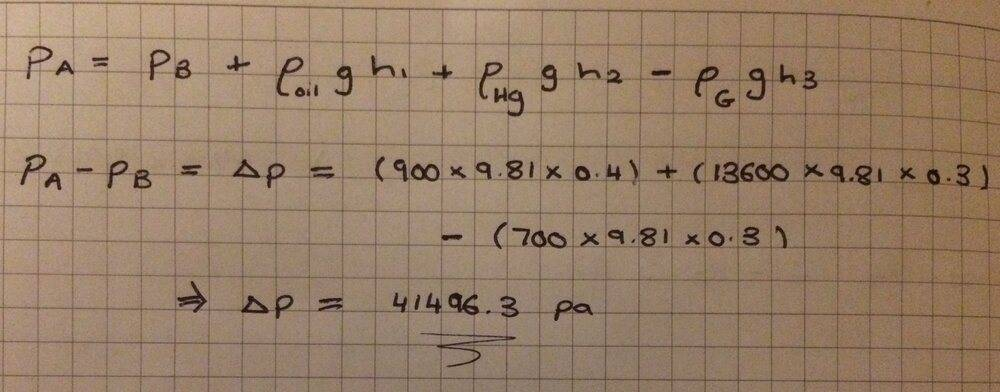Suppose the height difference goes from 0.3 m to 0.3 - 2x meters. In terms of x, what is the pressure difference?

Suppose the height difference goes from 0.3 m to 0.3 - 2x meters. In terms of x, what is the pressure difference?

Could I try something like this where I find the new pressure difference to be 66496.3 pa
then equal that to what my equation looked like in post #4. Only thing to notice is that since bulb A is exerting less pressure, mercury column on LHS would rise up and so we need to find by how much this new increased distance is. I called this x

Not sure if this is the right approach though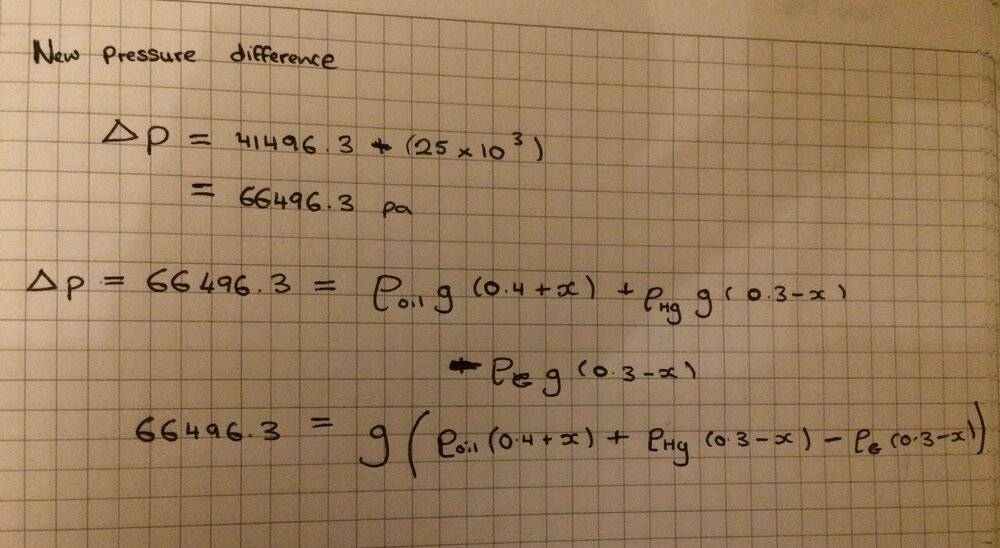Suppose the height difference goes from 0.3 m to 0.3 - 2x meters. In terms of x, what is the pressure difference?

why does the height difference change from 0.3 to 0.3 – 2x though?

The fluid level in the left arm moves up x, and the fluid in the right arm moves down x.
So the column of gasoline decreases to ...?
And the column of oil increases to ...?
And the difference in height of the mercury columns decreases to ...?

The answer to my question has to depend on x.

The fluid level in the left arm moves up x, and the fluid in the right arm moves down x.
So the column of gasoline increases to ...?
And the column of oil increases to ...?
And the difference in height of the mercury columns decreases to ...?

The answer to my question has to depend on x.

wait hold on if the fluid level in the left arm goes up
then the column of gasoline decreases to '0.3 - x' ?
and the column of oil increases to '0.4 + x'

and the difference in height of the mercury columns must decrease to '0.3 - 2x'

Am I right?

wait hold on if the fluid level in the left arm goes up
then the column of gasoline decreases to '0.3 - x' ?
and the column of oil increases to '0.4 + x'

and the difference in height of the mercury columns must decrease to '0.3 - 2x'

Am I right?
correct so far. So, now, in terms of x, what is the pressure difference PA-PB?

correct so far. So, now, in terms of x, what is the pressure difference PA-PB?
[/QU

I did this: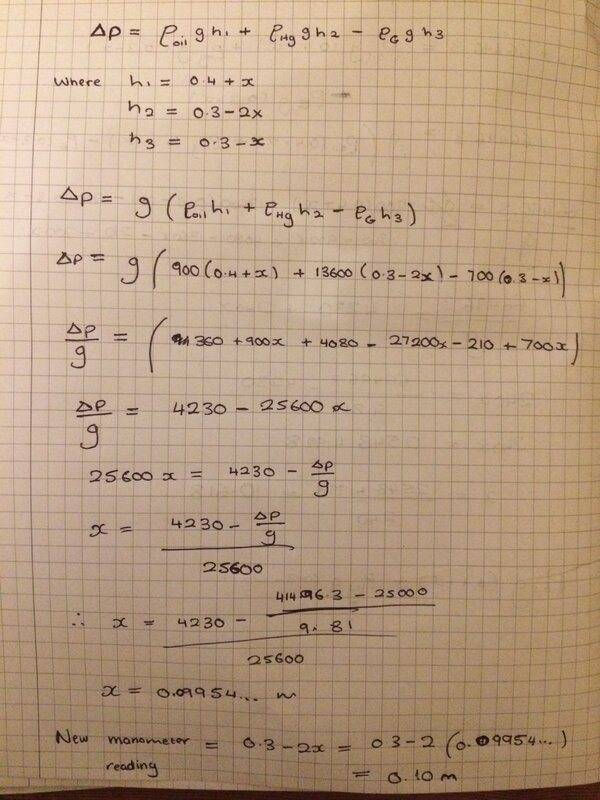I took pressure change to be 16496.3 pa (where I subtracted 25kpa from 41496.3 pa) and not 66496.3 pa (where I added 25kpa to 41496.3 pa) If I had used 66496.3 pa I would've got a negative x value

I got the final manometer reading to be 0.10m which is the right answer but my workings seems a bit long to get to this answer. Is this what you had meant express PA-PB (pressure difference) in terms of x?

Last edited:
Is there a more concise way to express pressure difference PA-PB in terms of x or should I stick to the one shown in post #11

Yes. My motivation was that, if you first figured out how to get the initial pressure difference, it would be easier to solve the problem. The rest seems pretty straightforward to me.

•Bolter